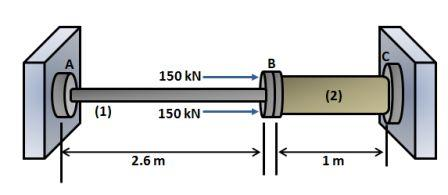# A solid aluminum \left ( E = 70 GPa; \alpha =22.5\times 10^{-6}/^{\circ}C \right ) rod (1) is...

## Question:

A solid aluminum {eq}\left [ E = 70 GPa; \alpha =22.5\times 10^{-6}/^{\circ}C \right ] {/eq} rod (1) is connected to a solid bronze {eq}\left [ E = 100 GPa; \alpha =16.9\times 10^{-6}/^{\circ}C \right ] {/eq} rod (2) flange B as shown. Aluminum rod (1) has an outside diameter of 46 mm and bronze rod (2) has an outside diameter of 144 mm. t The bars are unstressed when the structure is assembled at {eq}30^{\circ}C {/eq}. After the 300- kN loads is applied to flange B, the temperature increases to {eq}45^{\circ}C {/eq}.

Determine the normal force in rod (2).## Hooke's law:

Hook's law is the law which is valid in the elastic region where stress is directly proportional to strain upto elastic limit. The slope of the stress-strain diagram gives the value of the Young's Modulus for the given material.

## Answer and Explanation: 1

Become a Study.com member to unlock this answer! Create your account

Given Data

• The modulus of elasticity of aluminum rod: {eq}{E_{\rm{a}}} = 70000\;{\rm{MPa}} {/eq}.
• The coefficient of thermal expansion of aluminum...

See full answer below.

#### Learn more about this topic:Hooke's Law & the Spring Constant: Definition & Equation

from

Chapter 4 / Lesson 19
202K

After watching this video, you will be able to explain what Hooke's Law is and use the equation for Hooke's Law to solve problems. A short quiz will follow.